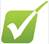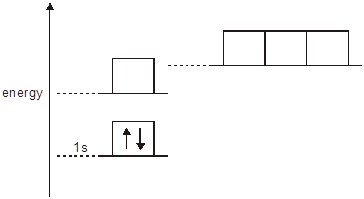Atomic structure review1)    Define the term first ionisation energy.  

2)    Electrons are arranged in energy levels.

Complete the table below to show how many electrons completely fill each of the following.  

 number of electrons a d orbital a p sub-shell the third shell (n = 3)

3)    The energy diagram below is for the eight electrons in an oxygen atom. The diagram is incomplete as it only shows the two electrons in the 1s level.

Complete the diagram for the oxygen atom by:

(a)      adding labels for the other sub-shell levels,  

(b)     adding arrows to show how the other electrons are arranged.4)    The table shows the first 6 successive ionisation energies of an element X, which is in Period 3 of the Periodic Table.

Use the table to identify element X. Explain how you decided on your answer.  

 element ionisation energy / kJ mol–1 1st 2nd 3rd 4th 5th 6th X 578 1817 2745 11 578 14 831 18 378

5)    (a)      Write an equation, with state symbols, to represent the second ionisation energy of calcium.  

(b)      Why is the second ionisation energy of calcium greater than their first ionisation energies?  

(c)     Explain why the first ionisation energy of strontium are less than that of calcium.  

6)    The electronic configuration of a bromine atom can be written in terms of sub-shells.

(a)      Complete the electronic configuration of a bromine atom.  

1s22s22p63s23p6 ...............

(b)      Why is bromine classified as a p-block element?  

(c)    Write the electron configuration for a bromine ion  

1s22s22p63s23p6 ...............